Developing TOPSIS method using statistical normalization for selecting Knowledge management strategiesDeveloping TOPSIS method using statistical normalization for selecting Knowledge management strategies

Amin Zadeh Sarraf, Ali Mohaghar, Hossein Bazargani

University of Tehran (Iran)

Accepted: July 2013

Zadeh Sarraf, A., Mohaghar, A., Bazargani, H. (2013). Developing TOPSIS method using statistical normalization for selecting Knowledge management strategies. Journal of Industrial Engineering and Management, 6(4), 860-875. http://dx.doi.org/10.3926/jiem.573

---------------------

Abstract:

Purpose: Numerous companies are expecting their knowledge management (KM) to be performed effectively in order to leverage and transform the knowledge into competitive advantages. However, here raises a critical issue of how companies can better evaluate and select a favorable KM strategy prior to a successful KM implementation.

Design/methodology/approach: An extension of TOPSIS, a multi-attribute decision making (MADM) technique, to a group decision environment is investigated. TOPSIS is a practical and useful technique for ranking and selection of a number of externally determined alternatives through distance measures. The entropy method is often used for assessing weights in the TOPSIS method. Entropy in information theory is a criterion uses for measuring the amount of disorder represented by a discrete probability distribution. According to decrease resistance degree of employees opposite of implementing a new strategy, it seems necessary to spot all managers’ opinion. The normal distribution considered the most prominent probability distribution in statistics is used to normalize gathered data.

Findings: The results of this study show that by considering 6 criteria for alternatives Evaluation, the most appropriate KM strategy to implement in our company was ‘‘Personalization’’.

Research limitations/implications: In this research, there are some assumptions that might affect the accuracy of the approach such as normal distribution of sample and community. These assumptions can be changed in future work

Originality/value: This paper proposes an effective solution based on combined entropy and TOPSIS approach to help companies that need to evaluate and select KM strategies. In represented solution, opinions of all managers is gathered and normalized by using standard normal distribution and central limit theorem.

Keywords: knowledge management; strategy; TOPSIS; Normal distribution; entropy

---------------------

1. Introduction

In the knowledge economy, it's necessary to find a way to create, share, and utilize knowledge if we want to have a competitive advantage (Desouza, 2003). Nowadays business environment has been more competitive, in such situation, many companies emphasize the importance of knowledge management (KM), and base the KM strategy on their unique resources and capabilities. According to (Kamara, Anumba & Carrillo, 2002), KM is the organizational optimization of knowledge to achieve enhanced performance through the use of various methods and techniques. Also, KM is a systemic way to manage knowledge in the organizationally specified process of acquiring, organizing, and communicating knowledge.

More importantly, the effective KM largely begins with a proper KM strategy. Hence, in order to implement the KM successfully, there is a critical issue of how companies can better evaluate and select a favorable KM strategy. However, the KM strategy selection usually involves subjective and qualitative judgment. In particular, choosing KM strategies is a strategic issue (Bierly & Chakrabarti, 1996), which is restricted by resource needs, realistic support, time requirements, and conformity with expected outcomes or business purposes. In this sense, the treatment of KM strategy selection is required to handle several complex factors in a better sensible and logical manner. Thus, the KM strategy selection is a kind of multiple criteria decision-making (MCDM) problem, and requires MCDM methods to solve it appropriately. Many traditional MCDM methods are based on the additive concept along with the independence assumption, but each individual criterion is not always completely independent (Leung, Hui & Zheng, 2003).For solving the interactions among elements, entropy as a relatively new MCDM method was proposed by Shannon (Shannon, 1948). Although entropy has been used in this article, TOPSIS presented by Hwang and Yoon (Hwang & Yoon, 1981) has been utilized for evaluation of alternatives. According to decrease resistance of employees against implementing a new KM strategy in our organization, we extended TOPSIS and formed62empty decision matrixes and distribute them between all managers of organization to cooperate in decision making process by fulfilling those matrixes. After gathering these completed matrixes, we should make a single matrix as final decision matrix for applying TOPSIS and entropy. To reach this purpose we combined results of these 62 matrix. This was by using arithmetic average of every cell's value in all decision matrixes completed by managers. In order to normalize decision matrix, normal distribution and central limit theorem has been utilized.

2. Literature survey

In this section, some essentials of the entropy and the TOPSIS are briefly described. A table that contained all normalization methods is represented too. At the end of this part a brief description about Normal distribution and Central limit theorem is represented.

2.1. Entropy

The entropy method can be used not only to quantitatively estimate data quantity, but also to calculate objectively the relative weight of information (Shannon, 1948). Entropy was originally intended to simplify a physical phenomenon of numerator turbulence degree or the probability scale under a specified condition. If entropy values are lower, the numerator degrees are more proportional, implying as close to perfect entropy as possible. Conversely, if entropy values are higher, the numerator degrees have a more irregular inflection. Therefore, entropy weight method was introduced to obtain the relative weight of each attribute. Additionally, in information theory, entropy can be used to measure expected information content of a certain message. Entropy in information theory is a criterion for the amount of uncertainty represented by a discrete probability distribution (Jaynes, 1957). Each attribute is assigned measured a value by each alternatives to calculate the entropy values. The entropy values for each criterion are then compared, and the relative significance levels of each other are calculated (i.e., the relative weight). Next, the entropy weight is obtained based on the appraisal matrix information, which belongs to the objective weight values. Calculation procedure for the entropy weight method has been described in part 3, evaluation framework.

2.2. TOPSIS

The TOPSIS method was first developed by Hwang and Yoon (Hwang & Yoon, 1981) and ranks the alternatives according to their distances from the ideal and the negative ideal solution, i.e. the best alternative has simultaneously the shortest distance from the ideal solution and the farthest distance from the negative ideal solution. The ideal solution is identified with a hypothetical alternative that has the best values for all considered criteria whereas the negative ideal solution is identified with a hypothetical alternative that has the worst criteria values. In practice, TOPSIS has been successfully applied to solve selection/evaluation problems with a finite number of alternatives (Jee & Kang, 2000; Yong, 2006) because it is intuitive and easy to understand and implement. Furthermore, TOPSIS has a sound logic that represents the rationale of human choice (Shih, Syur & Lee, 2007) and has been proved to be one of the best methods in addressing the issue of rank reversal (Zanakis, Solomon, Wishart & Dublish, 1998).In this paper we extended TOPSIS for KM strategies selection problem because of following reasons and advantages as Shih and his cooperators did for consultant selection problem (Shih et al., 2007).

• A sound logic that represents the rational of human choice.

• A scalar value that accounts for both the best and worst alternative simultaneously.

• A simple computation process that can be easily programmed into a spreadsheet.

• The performance measures of all alternatives on attributes can be visualized on a polyhedron, at least for any two dimensions.

2.3. Common methods of normalization for TOPSIS

For MADM, a decision matrix is usually required prior to the beginning of the process. The decision matrix contains competitive alternatives row-wise, with their attributes’ ratings. Normalization is an operation to make these scores conform to or reduced to a norm or standard. To compare the alternatives on each attribute, the normalized process is usually made column-wise, and the normalized value will be a positive value between 0 and 1. In this way, computational problems, resulting from different measurements in the decision matrix, are eliminated (Yoon & Hwang, 1995). Attributes have been partitioned into three groups: benefit attributes, cost attributes, and non-monotonic attributes (Hwang & Yoon, 1981). A few common normalization methods are organized in Table 1 (Milani, Shanian, Madoliat & Nemes, 2005; Hwang & Yoon, 1981; Yoon & Hwang, 1995). These are classified as vector normalization, linear normalization and fuzzy normalization to fit real-world situations under different circumstances. Additionally, three forms for linear normalization are listed in Table 1.

2.4. Normal distribution

In many applications in which some random variable X is normally distributed with mean µ and variance s2, we will standardize X to obtain z-scores (z=(x-m)/s2). The distribution of the z-scores is the standard normal distribution, that is, the normal distribution with a mean of zero and a variance of one.

Therefore, if X complies N(m, s2), then Z abides by N(0,1) also (Belsom, 1992). The probability density function of the standard normal distribution is as follows: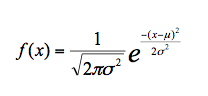The cumulative distribution function (CDF) of a probability distribution contains the probabilities that a random variable X is less than or equal to X. The cumulative distribution function of the normal distribution is expressed as follows:The normal distribution is considered the most prominent probability distribution in statistics. There are several reasons for this. One of them is that normal distribution is very tractable analytically, that is, a large number of results involving this distribution can be derived in explicit form (Casella & Berger, 1990).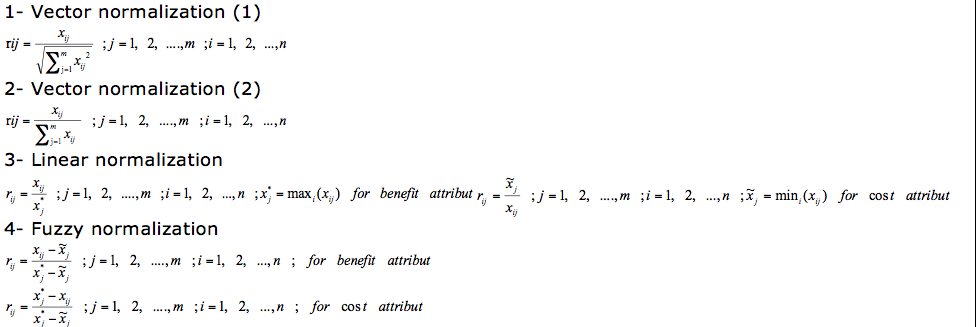Table 1. Common methods of normalization for TOPSIS

2.5. Central limit theorem

The importance of normal distribution as a model of quantitative phenomena in the natural and behavioral sciences is due to the central limit theorem. Under certain conditions (such as being independent and identically distributed with finite variance) the sum of a large number of random variables is approximately normally distributed, this is the central limit theorem. Many psychological measurements and physical phenomena (like noise) can be approximated well by the normal distribution. While the mechanisms underlying these phenomena are often unknown, the use of the normal model can be theoretically justified by assuming that many small, independent effects additively contribute to each observation.

Zhonggen devoted to the study of central limit theorems and the domain of normal attraction for some random processes with sample paths in exponential spaces under metric entropy conditions (Zhonggen, 1997).

Yokoyama studied on this line the functional central limit theorem and law of the iterated logarithm for stationary processes, not necessarily possessing the boundary decomposition, with applications to stationary linear processes (Yokoyama, 1995).

Dedecker and Prieur proved a central limit theorem for the d-dimensional distribution function of a class of stationary sequences(Dedecker & Prieur, 2007).

3. Evaluation framework

Selection of KM strategy is a kind of MCDM problem that needs multiple evaluation criteria. (Opricovic & Tzeng, 2004) represented that, MCDM problem solving is required to define evaluation criteria, find alternatives and evaluating of them in terms of criteria, apply an appropriate multi-criteria analysis method, and choose the best alternatives. Decision making is the process of defining the decision goals, gathering relevant criteria and possible alternatives, evaluating the alternatives for advantages and disadvantages, and selecting the optimal alternative (Hess & Siciliano, 1996).

In this paper, a new method of normalization has been represented. This method called statistical method for normalization is so applicable when our data has been selected from a normal statistical society. In fact when judgment about some alternatives is implemented by some persons and we selected average value of each element and create decision matrix based on them and want to evaluate alternatives according to this matrix, we can use this method for normalization of decision matrix. We use in this article consists of four steps. In the next section a brief description about each of these steps has been represented.

• Defining the problem objectives

• Defining alternatives and criteria for evaluating

• Applying ENTROPY model and TOPSIS

• Choosing the most appropriate strategy

3.1. Defining the problems objectives:

As mentioned in the past section, decision making is the process of defining the decision goals, gathering relevant criteria and possible alternatives, evaluating the alternatives for advantages and disadvantages, and selecting the optimal alternative (Hess & Siciliano, 1996). Each organization has it's own purpose by implementing KM strategies. For instance, KM is the way to improve an organization’s performance, productivity, and competitiveness and to promote learning, sharing, and usage of knowledge. The purpose of KM can be different such as: to initiate action based on knowledge; to support business strategy implementation; to become an intelligent enterprise; to increase competitive advantage; to create an innovative culture and environment; to entrench collaboration as a work practice; and to improve work efficiency (Plessis, 2005). In this phase, the objectives of our decision should become evident. Here is defining and choosing the appropriate KM strategy as defining the problem objectives in phase 1.

3.2. Defining alternatives and criteria for evaluating

Defining alternatives and criteria for evaluating of them is the second phase as gathering relevant criteria and possible alternatives. In selecting appropriate strategy for an organization, it is critical to form a team and involve several experts from different departments to create the best adaptability between organization and proposed strategies for organization (Saremi, Mousavi & Sanayei, 2009). It's very important to make an effective and efficient communication between different experts because the better the parties are informed about strategy selection, the higher the probability that the parties will be committed to supporting this selection, The more different perspectives are initially taken into account, and the greater the complexity of convergence, the smaller the chances of addressing the wrong problem and reaching an inadequate solution (Karacapilidis, Adamides & Evangelou, 2006).

The objectives of selection, the scope of selection, and the possible alternatives should become defined as well as possible. In this phase, after gathering data by Interviewing with elites, the data has been categorized, analyzed, and summarized to decision making matrix. As to alternatives of KM strategy, (Hansen, Nohria & Tierney, 1999) represented two types of KM strategies: the codification strategy (seeking to document and store knowledge in databases) and the personalization strategy (seeking to develop networks of people for communicating ideas).

In our research, the strategic management team of the organization defined third strategy as Blend strategy (a mixture between codification and personalization). Evaluation criteria of KM strategy can range from top management support, communication, creativity, culture and people, sharing knowledge, incentives, time, and evaluation (Martensson, 2000). Strategic management team of the organization represented that evaluation criteria of KM strategy can range from top management support, time, cost, degree of acceptance by employees, technical knowledge, and knowledge sharing. So, 6 criteria selected for evaluation of 3 chosen strategies.

At the end of this phase a set of possible alternatives for implemention in organization is prepared that we called them A = {A1, A2, …, Am}. Also, a set of necessary criteria selected that we called them C = {C1, C2, ..., Am}.

3.3. Applying entropy and TOPSIS methods

After defining alternatives and evaluation criteria, it is necessary to apply an entropy model and the TOPSIS; the entropy model is used to calculate the elements of evaluation criteria's weights, and the TOPSIS is used to solve problem and choose the best strategy for this organization. Here are the steps of applying entropy and TOPSIS on this problem.

3.3.1. Establish a decision matrix for alternative performance

According to select the best knowledge strategy for our organization, we formed strategic team from top managers of organization at first. Outcome of this team after forming several meeting, as mentioned in past paragraphs, was 3 strategy and 6 criteria for evaluation of them. After definition of alternatives and criteria, we must gather relevant data for these alternatives. According to this purpose, 62 empty decision matrix is included 3 rows and 6 columns formed and delivered to all managers of organization in strategic, middle and functional level. There were 62 managers in these levels in our organization. These managers evaluated different alternatives and completed these matrixes. In order to evaluation of results, we, as investigative teams, need just one matrix. As mentioned in the past paragraph that we had 62 matrixes, it was necessary to convert these matrixes to only one matrix. To reach this purpose, we formed an empty matrix by 3 rows and 6 columns and filled it's cells by using arithmetic average of that cell in all 62 matrixes by below form: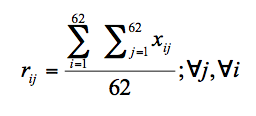Rij is the final value for each final decision matrix cells and xij is that cell value in 62 matrixes fulfilled by 62 managers.

The strategy selection problem can be expressed in the matrix format for k-th decision maker as follows:Where fij is a linguistic variable, indicates the performance rating of each ith alternative with respect to each jth criterion. In fact each element of final matrix is the average of that element in 62 primary decision matrixes. We have shown our alternatives as Si = {S1, S2, S3} and criteria as Cj = {C1, C2, C3} and the data belongs them in Table 2 as final decision matrix.

 C1 C2 C3 C4 C5 C6 S1 5.41 24 15,700 7.25 6.58 7.69 S2 8.32 12 6,400 3.5 4.12 3.87 S3 5.98 19 12,800 5.2 8.5 5.63

Table 2. Final decision matrix

3.3.2. Apply entropy method

Each criterion in a MADM methods needs a weight to show its important. There is some methods for dedication of weight to criterias. As mentioned in Literature, one of this methods that is often used for assessing weight in the TOPSIS method, is entropy (Starr & Zeleny, 1977). Entropy usually has been used as a criterion for measuring of represented disorder by a discrete probability distribution (Pratyyush & Jian-Bo, 1998). The assumption of entropy is that a wide data distribution shows more disorder than a packed distribution (Pratyyush & Jian-Bo, 1998). When we want to seek for contrast between sets of data, its useful to use entropy method. Here is the entropy steps and it's result on our decision making matrix:

(a) Normalization of original decision matrix as: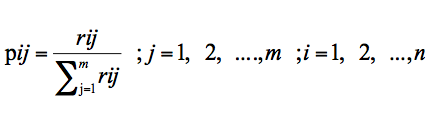The normalized form of our decision matrix has been shown in Table.3

 C1 C2 C3 C4 C5 C6 S1 0.27 0.44 0.45 0.45 0.34 0.45 S2 0.42 0.22 0.18 0.22 0.21 0.23 S3 0.30 0.35 0.37 0.33 0.44 0.33

Table 3. Normalized decision matrix

(b) Calculate the entropy of data for each criterion:

The entropy of the set of normalized outcomes of the jth criterion in the decision matrix is given by: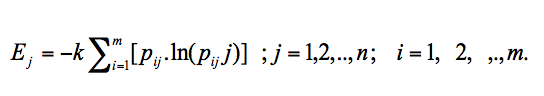k is a constant (normalizing) value and taken to be 1 = Ln(m). Note that if all normalized values fora criterion become identical, pij =1/m, and thus Ej = 1.

(c) Calculate the weights based on the entropies as follows: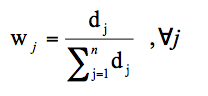Where, Dj = 1 – Ej, and it is the degree of diversity of the information involved in the outcomes of the jth criterion. If the decision maker (DM) wants to add his/her subjective weights according to experience, particular constraints or expectations and so on, the above weights are modified (Yoon & Hwang, 1980). We face such situation in our problem. When elites of our organization faced with the entropy's weights, they recognized that these weight are not appropriate for criteria, so they merged and modified their desirable weight with entropy weight with the below formula:Table 4 showes final matrix that include Ej , Dj , Wj , W' and lj.

 C1 C2 C3 C4 C5 C6 Ej 1.188 1.166 1.141 1.161 1.162 1.166 Dj -0.187 -0.166 -0.141 -0.161 -0.162 -0.166 Wj 0.191 0.169 0.143 0.164 0.165 0.169 lj 0.209 0.127 0.143 0.252 0.075 0.194 W' 0.237 0.127 0.122 0.245 0.074 0.195

Table 4. Final matrix

3.3.3. Apply TOPSIS method

Yoon and Hwang introduced the TOPSIS method based on the idea that the best strategy should have the shortest distance from an ideal solution. They assumed that if each attribute takes a monotonically increasing or decreasing variation, then it is easy to define an ideal solution (Yoon & Hwang, 1980). The steps of TOPSIS solution method and its results are shown as bellow:

(a) Normalization of the decision matrix

In this article we normalized decision matrix by a using concepts of Normal distribution. In fact, we represent the statistical normalization method. Here is definition of the steps of this method with it's result on our decision matrix:

(a.1). Calculation of Z parameter:

It's obvious that normal distribution convert basic value of different statistics to standard value between -3.59 and +3.59 by decreasing mean of meter and dividing the result of this function on the standard deviation of data as show below formula: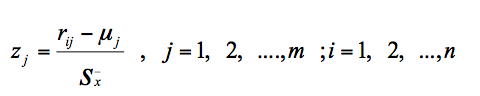Zij is the standard value of each data, mj is more favorable and rational content of each criterion that has been defined by experts of organization and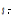is standard deviation of each criterion that calculates by below formula:N is number of alternative or in another hand, number of data for each criteria. Here is Z matrix in Table.5 that contains Z value of each data.

 C1 C2 C3 C4 C5 C6 S1 -0.92 1.15 1.05 1.26 0.10 1.26 S2 1.39 -1.29 -1.35 -1.18 -1.27 -1.19 S3 -0.47 0.14 0.30 -0.08 1.17 -0.06

Table 5. Z matrix

(a.2) Calculation of probability matrix:

After calculating standard value for each parameter by using standard distribution formula, it's time to calculate the probability of occurrence of standardized content. In this part we apply the below formula to obtain probability of occurrence of each criteria. For example, when we convert the content of first strategy (codification strategy) for first criterion (top management support) to probability of their occurrence, we actually calculated how much percent top management of organization support from implementing codification strategy and so on.Zij is the standard value for each rij.

Also we can use normal distribution table and calculate Probability of any standard content. it's important to mention that after such convert, the value of all content will become between 0 and 1 and in this time, we can continue extant steps of TOPSIS. Table 6 shows matrix of probabilities.

 P1 P2 P3 P4 P5 P6 S1 0.1778 0.8749 0.8531 0.8962 0.5398 0.8962 S2 0.9177 0.0985 0.0885 0.119 0.102 0.117 S3 0.3192 0.5557 0.6179 0.4681 0.879 0.4761

Table 6. Matrix of probabilities

(b) Multiply the columns of normalized decision matrix by the associated weights from entropy method. The weighted and normalized decision matrix is obtained as: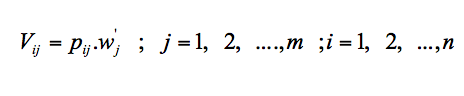W'j represents the weight of the jth attribute. Weighted and normalized decision matrix is shown in Table 7 as below:

 V1 V2 V3 V4 V5 V6 S1 0.042 0.111 0.104 0.220 0.040 0.175 S2 0.217 0.013 0.011 0.029 0.008 0.023 S3 0.076 0.071 0.075 0.115 0.065 0.093

Table 7. Weighted and normalized decision matrix

(c) Determine the ideal and nadir ideal solutions. The ideal values set and the nadir values set are determined as follows: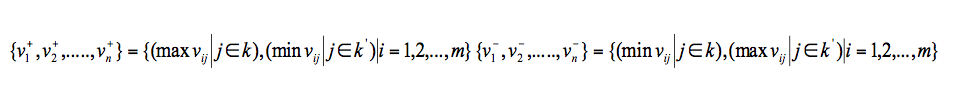Where, K is the index set of benefit criteria and K' is the index set of cost criteria.

(d) Measure distances from the ideal and nadir solutions. The two Euclidean distances for each alternative are calculated as:(e) Calculate the relative closeness to the ideal solution. The relative closeness to the ideal solution can be determined as:We calculated the content of ideal and nadir ideal, distances of each alternative from the ideal and nadir for our problem, and the relative closeness to the ideal solution and represent results in Table 8.

 S+ S- C S1 0.22404106 0.24496318 0.52230483 S2 0.243683 0.22898631 0.48445352 S3 0.22052238 0.12579726 0.36324032

Table 8. Relative closeness to the ideal solution

According to Table 8, because of the highest grant for strategy number 1 (personalization strategy) as relevant closeness grant, it is the most appropriate strategy for Implementation in our organization.

4. Conclusion

As knowledge is taking on an important strategic role, numerous companies are expecting their KM to be performed effectively in order to leverage and transform the knowledge into competitive advantages. More importantly, the successful KM starts with a proper KM strategy that is produced through a robust evaluation method. However, the KM strategy selection is a kind of MCDM problem, which requires considering a large number of complex factors as multiple evaluation criteria. Although numerous creditable works are devoted to the study of how to build a KM strategy and to execute the KM successfully, few of those have provided methods which can systematically evaluate and model complex factors of the KM strategy.

Dealing with the MCDM problem of this KM strategy selection, it is better to employ MCDM methods for reaching an effective problem-solving. The entropy method is useful because of high capability of this method in estimating weights. It's important to alert that when criteria for evaluation of some alternative have interdependence relation with each other, we can't define criteria's weight without any attention to the other criteria and their effects on each other. Moreover, the TOPSIS not only can be used as a way to handle the inner dependences within a set of criteria, but also can produce more valuable information for making decisions. Hence, this paper proposes a solution based on a combined entropy and TOPSIS approach to help companies needed to evaluate and select KM strategies.

It's important to note establishing a new technology or implementing a new strategy such as KM strategy always has a degree of resistance by employees. For decreasing this resistance, we use an extension of TOPSIS for group thinking, want every manager of organization to evaluate 3 alternatives by 6 criteria, and finally put average of each parameter in final decision matrix.

Because of using average of each parameter as basic data, we use central limit theorem and normal distribution for standardizing data.

The results of this study show that the most appropriate KM strategy was ‘‘Personalization’’. Because the proposed solution can handle the effects of dependences, it is relatively useful and makes the evaluation result to be more reasonable. Additionally, this study has contributed to extend practical applications of both entropy and TOPSIS in KM field.

Furthermore, using the suggested statistical procedure for normalization, can effectively handle any problem of selection with multi-decision matrix.

References

Belsom, C. (1992). The Normal Distribution. The School Mathematics Project 16-19. Cambridge: Cambridge University Press.

Bierly, P., & Chakrabarti, A. (1996). Generic knowledge strategies in the US pharmaceutical industry. Strategic Management Journal, 17(4), 123-135. http://cat.inist.fr/?aModele=afficheN&cpsidt=2592598

Casella, G., & Berger, R.L. (1990). Statistical Inference. Belmont, CA: Duxbury Press.

Dedecker, J., & Prieur, C. (2007). An empirical central limit theorem for dependent sequences. Stochastic Processes and their Applications, 117, 121-142. http://www.sciencedirect.com/science/article/pii/S0304414906000895 http://dx.doi.org/10.1016/j.spa.2006.06.003

Desouza, K.C. (2003). Strategic contributions of game rooms to knowledge management: Some preliminary insights. Information and Management, 41(1), 63-74. http://www.sciencedirect.com/science/article/pii/S0378720603000272 http://dx.doi.org/10.1016/S0378-7206(03)00027-2

Hansen, M.T., Nohria, N., & Tierney, T. (1999). What’s your strategy for managing knowledge? Harvard Business Review, 77(2), 106-126.

Hess, P., & Siciliano, J. (1996). Management: Responsibility for performance. New York: McGraw-Hill.

Hwang, C.L., & Yoon, K. (1981). Multiple attribute decision making: Methods and applications. Heidelberg: Springer. http://dx.doi.org/10.1007/978-3-642-48318-9

Jaynes, E.T. (1957). Information theory and statistical mechanics. Physical Review, 106(4), 620-630. http://www.weizmann.ac.il/complex/tlusty/courses/InfoInBio/Papers/JaynesInformationTheory.pdf http://dx.doi.org/10.1103/PhysRev.106.620

Jee, D.H., & Kang, J.K. (2000). A method for optimal material selection aided with decision making theory. Materials and Design, 21(3), 199-206. http://dx.doi.org/10.1016/S0261-3069(99)00066-7

Kamara, J.M., Anumba, C.J., & Carrillo, P.M. (2002). A clever approach to selecting a knowledge management strategy. International Journal of Project Management, 20(3), 205‑211. http://dx.doi.org/10.1016/S0263-7863(01)00070-9

Karacapilidis, N., Adamides, E., & Evangelou, C. (2006). A computerized knowledge management system for the manufacturing strategy process. Computers in Industry, 57, 178-188. http://www.sciencedirect.com/science/article/pii/S0166361505001399 http://dx.doi.org/10.1016/j.compind.2005.07.001

Leung, L.C., Hui, Y.V., & Zheng, M. (2003). Analysis of compatibility between interdependent matrices in ANP. Journal of the Operational Research Society, 54(7), 758-768. http://www.palgrave-journals.com/jors/journal/v54/n7/full/2601569a.html http://dx.doi.org/10.1057/palgrave.jors.2601569

Martensson, M. (2000). A critical review of knowledge management as a management tool. Journal of Knowledge Management, 4(3), 204-216. http://www.emeraldinsight.com/journals.htm?issn=1367-3270&volume=4&issue=3&articleid=883705&show=abstract http://dx.doi.org/10.1108/13673270010350002

Milani, A.S., Shanian, A., Madoliat, R., & Nemes, J.A. (2005). The effect of normalization norms in multiple attribute decision making models: A case study in gear material selection. Structural Multidisciplinary Optimization, 29(4), 312-318. http://dx.doi.org/10.1007/s00158-004-0473-1

Opricovic, S., & Tzeng, G.H. (2004). Compromise solution by MCDM methods: A comparative analysis of VIKOR and TOPSIS. European Journal of Operational Research, 156(2), 445-455. http://dx.doi.org/10.1016/S0377-2217(03)00020-1

Plessis, M. (2005). Drivers of knowledge management in the corporat environment. International Journal of Information Management, 25(3), 193-202. http://dx.doi.org/10.1016/j.ijinfomgt.2004.12.001

Pratyyush, S., & Jian-Bo, Y. (1998). Multiple Criteria Decision Support in Engineering Design. Berlin: Springer Verlag.

Saremi, M., Mousavi, S.F., & Sanayei, A. (2009). TQM consultant selection in SMEs with TOPSIS under fuzzy environment. Expert Systems with Applications, 36, 2742-2749. http://dx.doi.org/10.1016/j.eswa.2008.01.034

Shannon, C.E. (1948). The mathematical theory of communication. Bell System Technical Journal, 27, 379-423. http://dx.doi.org/10.1002/j.1538-7305.1948.tb01338.x

Shih, H.S, Syur, H.J, & Lee, E.S. (2007). An extension of TOPSIS for group decision making. Mathematical and Computer Modeling, 45, 801-813. http://dx.doi.org/10.1016/j.mcm.2006.03.023

Starr, M.K., & Zeleny, M. (1977). Multiple Criteria Decision Making. New York: North Holland Publication Company.

Yokoyama, R. (1995). On the central limit theorem and law of the iterated logarithm for stationary processes with applications to linear processes. Stochastic Processes and their Applications, 59, 343-351. http://dx.doi.org/10.1016/0304-4149(95)00038-9

Yong, D. (2006). Plant location selection based on fuzzy TOPSIS. International Journal of Advanced Manufacturing Technology, 28(7-8), 839-844. http://dx.doi.org/10.1007/s00170-004-2436-5

Yoon, K.P., & Hwang, C.L. (1995). Multiple Attribute Decision Making: An Introduction. Thousand Oaks, CA: Sage Pub.

Yoon, K., & Hwang, C.L. (1980). Multiple Attribute Decision Making Methods and Applications. A State of the Art Survey. Berlin: Springer Verlag.

Zanakis, S.H., Solomon, A., Wishart, N., & Dublish, S. (1998). Multi-attribute decision making: A simulation comparison of select methods. European Journal of Operational Research, 107(3), 507-529. http://dx.doi.org/10.1016/S0377-2217(97)00147-1

Zhonggen, S. (1997). Central limit theorems for random processes with sample paths in exponential Orlicz spaces. Stochastic Processes and their Applications, 66, 1-20. http://dx.doi.org/10.1016/S0304-4149(96)00117-2